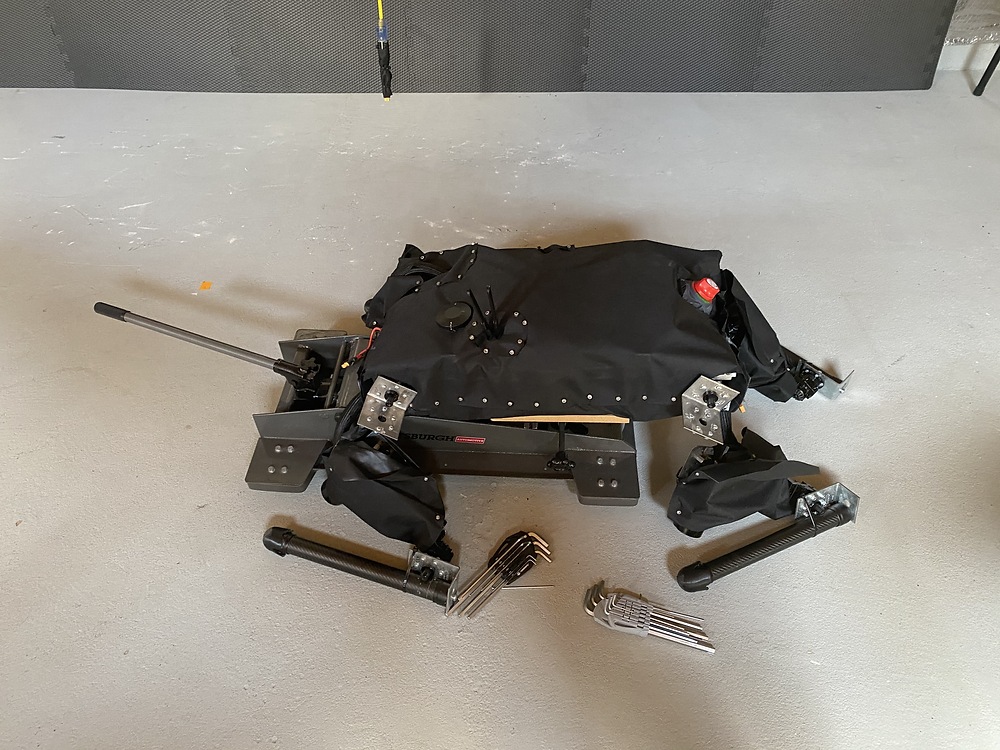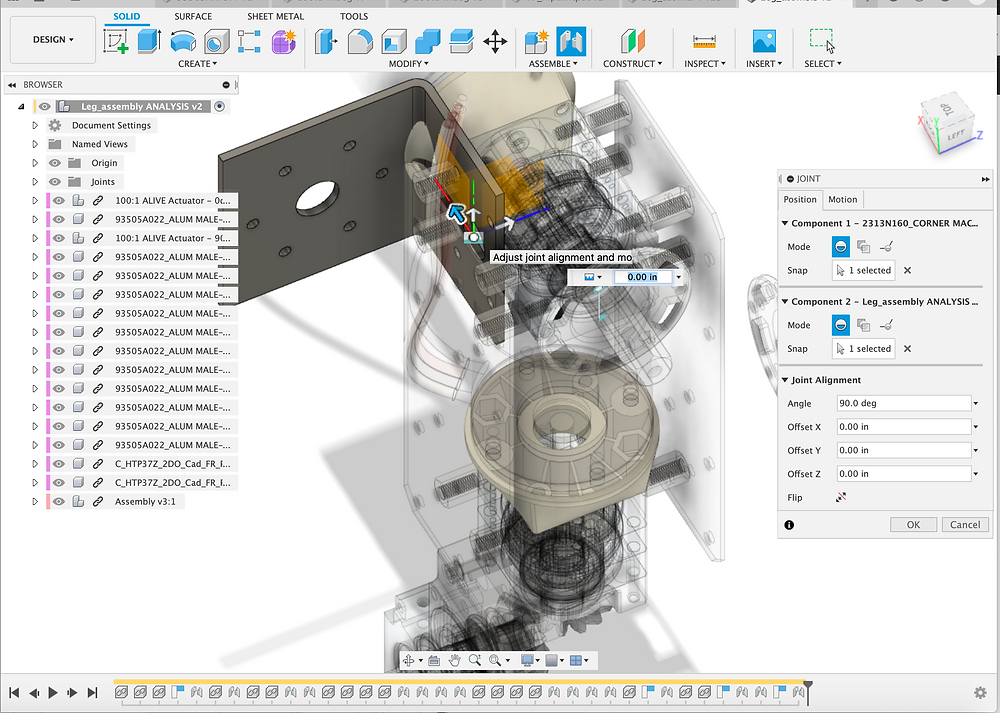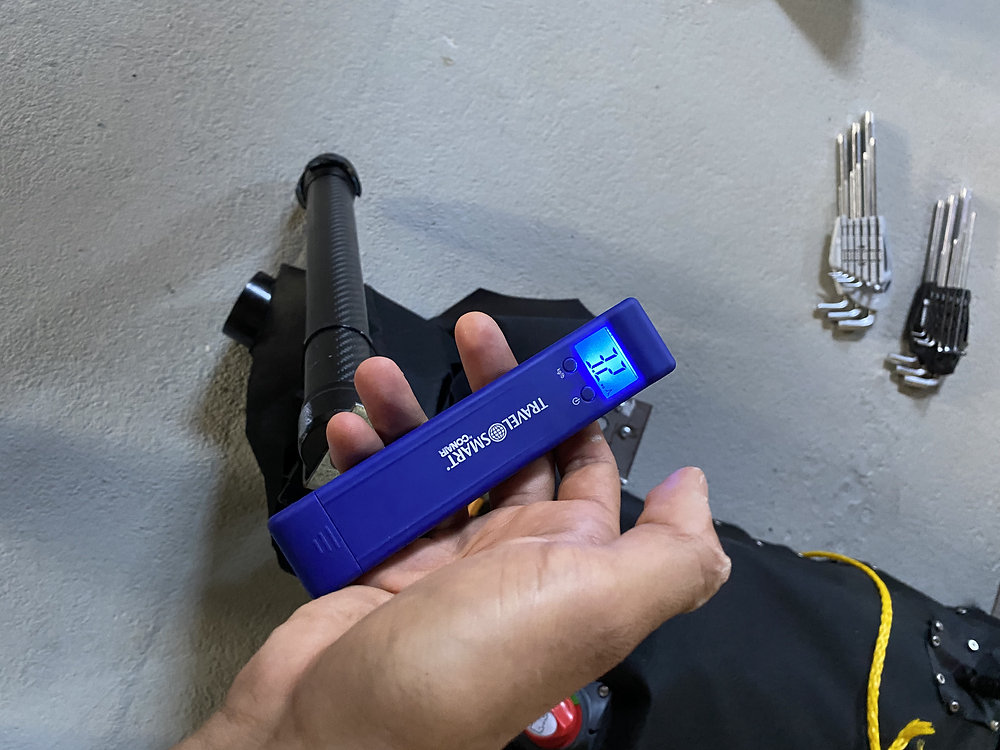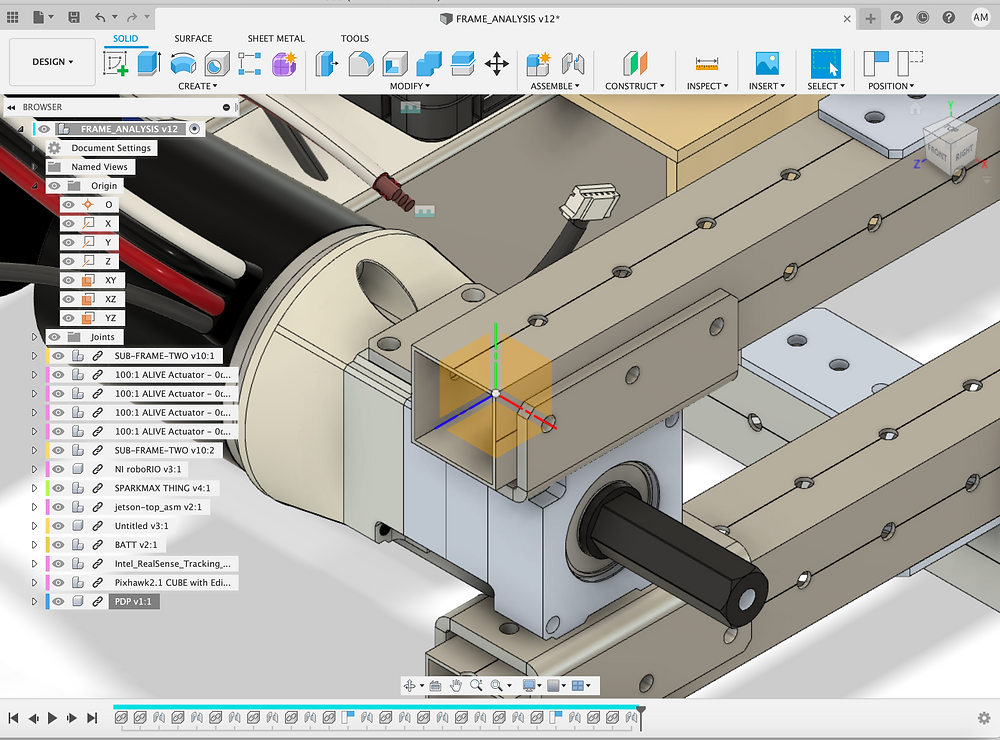Search
• aditya mehrotra.

# scout updates: quantifying the CoG of the body itself and the SLOP - INERTIAL MATRIX [updates]

So to be able to do some controls with this scout to actually try to make the robot walk we need to quantify a few things. We kind of want to quantify assuming there are no lies on the robot and the legs are mass-less (which they aren't but we need to know the CoG of at least the body itself for control), and we want to quantify (because slop is becoming an issue) what is the actual quantity of slop in degrees or number of rotations that we can see at the motor. We're going to use a digital pitch gauge to measure this one.

First: Let's start by measuring the quantity of slop we can see in one motor.

To quantify the slop in a single motor we're going to measure the reference position of a leg against one side of the slop in the gearbox then move it to the other side and measure the difference in what the pitch gauge is reading. The pitch gauge is accurate to +/- 0.05 deg.So here's the thing, that's -5.8deg --> 3.1deg which is a total of like 8.9deg +/- 0.1 deg! That's a large large amount of slop so what that means is we think we can be up to ~2.5 rotations of the motor OFF when we set the position of the leg. This will be much larger in the knee than in anywhere else because of the bevel gear in the knee joint.

We're going to now measure the slop in the upper gearboxes that don't have the bevel gear to figure out what the quantity of slop might be there so let's measure that. And that measurement is 16.4deg - 19.8deg which means the upper motors without the bevel gears have 3.4deg of slop. This might be insignificant compared to the 9deg of slop that come standard with the bevel gear setup. So those are the measurements.

```
Slop Measurement Results:

for knee w/ bevel gear = 8.9 deg +/- 0.1 deg
for all other actuators = 3.4 deg +/- 0.1 deg

```

So that leaves us with a max of 3.5deg and 9deg of slop in the shoulder joints and knee joint respectively. The higher end is chosen as a worst-case scenario and because the gauge was learning more on the higher than the lower side.

First Steps: Preparing the body and the legs for measurements.

So now we need to know what forces are on the body so we can predict what is going to happen to the platform in general. Right? So that includes the forces of gravity on the body and the force and torque from the legs. The first steps to this are to measure the CoG and the weight of just the body portion by removing the legs and etc. This should be fun and it'll likely take some time because we need to remove all four of the legs, but thanks to the simple design, removing the legs should be easy - just pop the collar off.Now we have a problem because one of the shaft collars on the front right leg - the one we actually care about has a stripped screw on it. So we might need to drill it out and replace the shaft collar, or at the very least the screw. So we're going to extract that collar and measure in the meantime we'll get some new screws. We'll do this by simply drilling.Now all four legs are off now that the screw has been drilled out. To do this you just take a drill bit of the same size as the head of the screw and then drill at it until it pops off. We saw the clamping collar actually pop off the shaft. We'll need to wait for the new one to come by before re-replacing it but that gives us a few days to do some measurements.So I know the gauge in the picture says 14.6 but I think that's because it was at the wrong place. WE moved it forward and took two other readings and both said 13.8kgs so that's what I think we're going to go with let's just double check. It's actually around like 13kgs, I think we pulled up too hard on the scale but we want to check a few times:

```
Measurement 1: 14.6
Measurement 2: 13.8
Measurement 3: 13.8
--THESE MEASUREMENTS ARE STANDARD AFTER THIS--
Measurement 4: 11.7
Measurement 5: 13.7
Measurement 6: 13.8
Measurement 7: 13.4

```

This data can be verified when we measure the weight of a leg and subtract the total known weight minus 4 legs. According to: https://www.adim.io/post/chip-updates-preliminary-experiments-with-weight-cog-motion-analysis-updates we get 24.8kgs of weight in total.

We did the same thing with just the leg and got about 0.8kgs! Note none of these measurements include the wires.

MOI and Mass in Fusion 360:

Now there's a few other things I just realized. We can directly measure things like MASS and etc, but we cannot directly measure things like the MoI. So what we really should do is go into Fusion 360. Make sure all the materials are the right material, and then we can run MASS and MoI analysis on all of them! We can even calculate the reflected MoI on the end of the gearbox from knowing the Neo motor's internal inertia. The NEO Motor has an estimated inertia of: 6.660E-05 kg*m^2...

The point of this though is we can now open Fusion 360, define the materials in the assembly a little more and start finding things such as the CoM, the moment of inertia about the CoM and the origin. And we can start comparing masses to the basses we are getting above. We know the total mass of most of the parts individually, so now it's time to match them in Fusion to find the MoI which will be helpful for control. We also need to add in a few thing to top-level. So here's what we know:

The aluminum frame is: 6061-T6 Aluminum

The aluminum mounts are: 5052 Aluminum

Gears: Rockwell C 55-62 Case Hardened Steel

Gearbox Housing: 6061-T6 Aluminum

Shafts: 7075-T6 Aluminum

Aluminium Standoffs: Aluminum

McMaster Angle Brackets: Stainless Steel

CIM Adapter: Glass Filled Nylon

Spacers: Acrylic

So starting with the frame and the sub-frame this is now what everything looks like:Now we move onto the gearboxes... the materials are defined above but let's actually make them right in the CAD.Now that all of these things have been updated we can turn out attention to the CoM and the MoI of the upper leg. We will ignore the lower leg because it's just a carbon fiber rod and we'll take the inertia of that as the reflected inertia of the motor through the gearbox for the knee joint let's see what mass we get. The CoM, and the MoI about x, y, and z.But the first thing we're going to do is move the bracket that connects the leg to the motor on the frame to the origin. And the reason we're going to do that is the software will give us the MoI about the origin and we really want to know the MoI about the point of rotation right?

```
Leg_assembly ANALYSIS v3

Component Instances (1)
Area	575.207 in^2
Density	3.686 ouncemass / in^3
Mass	168.019 ouncemass
Volume	45.577 in^3
Physical Material	(Various)

Bounding Box
Length 	 13.384 in
Width 	 11.793 in
Height 	 5.894 in
World X,Y,Z	0.00 in, 0.00 in, 0.00 in
Center of Mass	4.071 in, -2.131 in, -1.549 in

Moment of Inertia at Center of Mass   (ouncemass in^2)
Ixx = 1330.455
Ixy = -340.98
Ixz = 13.03
Iyx = -340.98
Iyy = 783.345
Iyz = -132.798
Izx = 13.03
Izy = -132.798
Izz = 1868.322

Moment of Inertia at Origin   (ouncemass in^2)
Ixx = 2496.704
Ixy = 1116.984
Ixz = 1072.464
Iyx = 1116.984
Iyy = 3971.405
Iyz = -687.408
Izx = 1072.464
Izy = -687.408
Izz = 5416.611

```

And a picture to note what the coordinate frame actually is - red is X, green is Y, blue is Z:Now that we know where the CoM is compared to the point of rotation of the leg, and we know the MoI with respect to the CoM as well as the joint location. We want to do the same thing to the main body but FIRST, we want to compare the mass of this to the mass we found by weighing the system.

Okay so we do have some discrepancy here... the total mass it is say is about 4kgs. Which is very consistent with the FIRST measurements but we really should re-measure this thing to see if it really is that heavy. The NEO Motor in the assembly is saying 1350g, yea and it's supposed to be: 0.4263768kgs so that's too heavy let's fix that. Now we've gotten that down to 464g (changed the material to aluminum).

So now this thing is 105 ounces which is: 2.9767 kgs. Now we want to really verify this with the other components. Let's go verify the leg's weight using the mass scale.Ah okay this makes more sense (yes this is one not very careful measurement) but I can believe 2.9kgs now because the CAD weight does not include the lower leg and this is the whole leg mass. So this makes a good amount of sense for Mass.

```
Leg_assembly ANALYSIS v5

Component Instances (1)
Area	575.207 in^2
Density	2.234 ouncemass / in^3
Mass	101.81 ouncemass
Volume	45.577 in^3
Physical Material	(Various)

Bounding Box
Length 	 13.384 in
Width 	 11.793 in
Height 	 5.894 in
World X,Y,Z	0.00 in, 0.00 in, 0.00 in
Center of Mass	3.594 in, -2.787 in, -1.611 in

Moment of Inertia at Center of Mass   (ouncemass in^2)
Ixx = 1041.05
Ixy = -127.055
Ixz = 12.13
Iyx = -127.055
Iyy = 389.989
Iyz = -113.864
Izx = 12.13
Izy = -113.864
Izz = 1249.568

Moment of Inertia at Origin   (ouncemass in^2)
Ixx = 2096.096
Ixy = 892.752
Ixz = 601.794
Iyx = 892.752
Iyy = 1969.647
Iyz = -571.052
Izx = 601.794
Izy = -571.052
Izz = 3355.57
```

Okay so here are the numbers for MoI and CoG for the upper part of the leg, now we're going to BOX this off and do the same for the lower body. But first we need to add components like the RoboRIO, Spark Max, the buttons, the Jetson TX1, etc.

Doing the Same for the Body:

So let's first list all the things we have to add to get this thing working. So we need:

• RoboRIO - 330 g

• Spark Max (12x) - 0.25lbs (113.3g)

• Jetson - 0.33

• Ardupilot - 0.1

• Intel Realsense - 55 g

• Batteries - 1.244

• Power Distribution Board - 6.06 x 5.98 x 1.93 inches and 11.4 ounces

• Power Converter + Button - 0.8391459

So now we need to CAD and insert these into the frame analysis CAD so let's do that. The total mass right now without the above components is 177oz = 5kgs. With the above it will be... 9.969kgs --> that makes sense for this because there are no wires and there is no nice cloth bag surrounding everything. So we'll make up for it with a ring around the center that weights like 0.898112893 kgs because that's about what the wires weigh. Let's get to CAD!

So we downloaded the files for the first 5, now we need to CAD the batteries, the wires, the PDP, and the Power Button. And it's time to create those CAD files now since we have finished editing the parts. Actually we downloaded two batteries too so we just need to CAD the distribution board and the power button. So I think we have all the parts now let's assemble them. Now I think we have stuck in everything:Now we're going to let Fusion sit and calculate things like the CoG and the MoIs. We'll display the origin for reference and etc. Here is the data and we're going to try to match the total mass: 11.4319452 kg is what we are measuring and we lifted it at 11.7-13.8 g so I think we're in the ballpark for a good estimate.

```
FRAME_ANALYSIS v12

Component Instances (1)
Area	4295.079 in^2
Density	1.57 ouncemass / in^3
Mass	403.253 ouncemass
Volume	256.821 in^3
Physical Material	(Various)

Bounding Box
Length 	 15.972 in
Width 	 7.499 in
Height 	 27.505 in
World X,Y,Z	0.00 in, 0.00 in, 0.00 in
Center of Mass	-5.782 in, 1.152 in, -10.585 in

Moment of Inertia at Center of Mass   (ouncemass in^2)
Ixx = 2.582E+04
Ixy = -9.361
Ixz = 84.777
Iyx = -9.361
Iyy = 2.886E+04
Iyz = 718.107
Izx = 84.777
Izy = 718.107
Izz = 4914.487

Moment of Inertia at Origin   (ouncemass in^2)
Ixx = 7.154E+04
Ixy = 2677.669
Ixz = -2.460E+04
Iyx = 2677.669
Iyy = 8.753E+04
Iyz = 5637.412
Izx = -2.460E+04
Izy = 5637.412
Izz = 1.893E+04

```Now we need to move the whole assembly down to the origin so we have a CoM reference from a KNOWN point that we can actually measure easily. We'll pick the upper-front-left corner of the frame and now we can move these distances.So now we can find the CoG, we can find the MoIs and we can compare these things to what we predicted and then we can start using all of this when we program the robot or do some calculations. This is very IMPORTANT because we might start training the robot using GAZEBO and it would be really important to know things like the inertial matrix and etc. We might be able to do some sort of verification of MoI by hand as well.

```
FRAME_ANALYSIS v13

Component Instances (1)
Area	4295.079 in^2
Density	1.57 ouncemass / in^3
Mass	403.253 ouncemass
Volume	256.821 in^3
Physical Material	(Various)

Bounding Box
Length 	 15.972 in
Width 	 7.499 in
Height 	 27.505 in
World X,Y,Z	0.00 in, 0.00 in, 0.00 in
Center of Mass	-6.55 in, -1.848 in, -12.085 in

Moment of Inertia at Center of Mass   (ouncemass in^2)
Ixx = 2.582E+04
Ixy = -9.361
Ixz = 84.777
Iyx = -9.361
Iyy = 2.886E+04
Iyz = 718.107
Izx = 84.777
Izy = 718.107
Izz = 4914.487

Moment of Inertia at Origin   (ouncemass in^2)
Ixx = 8.610E+04
Ixy = -4889.225
Ixz = -3.184E+04
Iyx = -4889.225
Iyy = 1.051E+05
Iyz = -8285.766
Izx = -3.184E+04
Izy = -8285.766
Izz = 2.359E+04
```

Okay so now we have a few of the major pieces of the model we are looking to create:

• First, we have the mass, the CoG, the MoI of the upper leg.

• Second, we have the mass, the CoG, the MoI, of the body.

• Third, we have the reflected Inertia form the actuators itself = 6.660E-05 kg*m^2 *100 *100 = 6.660E-01 kg*m^2.

• Fourth, we have quantified the slop in each of the joints, honestly the best way to deal with this is likely to just get rid of it on the physical system but you know.

We plan to end up turning all this data into a model that we can use to build a robot in Gzebo or at the very least, export STLs from Fusion 360 to import and assemble in Gazebo. We'll have to look into that more and also, switch computers bc Gazebo refuses to install on my Mac :(

This is a lot of really really good data to have and hopefully with some simulation in Gazebo if we start getting similar results to what we're getting in the real world, we might be able to use reinforcement learning to teach our dog how to walk properly who knows!

More info coming soon then :)

4 views0 comments

### Recent Posts

See All

#### chip updates: combining a kangaroo, a ship pulley, and a wild robot arm into a leg [updates]

© copyright 2019 | aditya mehrotra